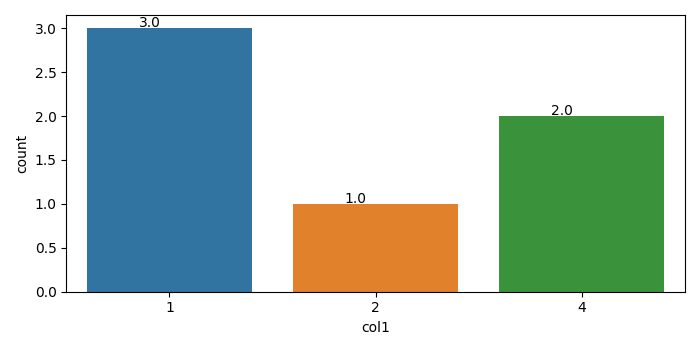# Matplotlib – How to show the count values on the top of a bar in a countplot?

MatplotlibPythonData Visualization

To show the count values on the top of a bar in a countplot, we can take the following steps

## Steps

• Set the figure size and adjust the padding between and around the subplots.

• Create a Pandas dataframe with one column.

• A countplot can be thought of as a histogram across a categorical, instead of a quantitative, variable.

• Iterate the returned axes of the countplot and show the count values at the top of the bars.

• To display the figure, use Show() method.

## Example

import seaborn as sns
import matplotlib.pyplot as plt
import numpy as np
import pandas as pd

plt.rcParams["figure.figsize"] = [7.00, 3.50]
plt.rcParams["figure.autolayout"] = True

df = pd.DataFrame(dict(col1=np.array([2, 4, 1, 1, 1, 4])))

ax = sns.countplot(x="col1", data=df)

for p in ax.patches:
ax.annotate('{:.1f}'.format(p.get_height()), (p.get_x()+0.25, p.get_height()+0.01))

plt.show()

## Output

It will produce the following output −